# Hypotenuse

Calculate the length of the hypotenuse of a right triangle with a catheti 71 cm and 49 cm long.

c =  86.267 cm

### Step-by-step explanation: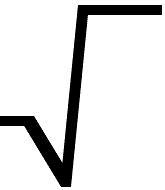Did you find an error or inaccuracy? Feel free to write us. Thank you!Tips to related online calculators
Pythagorean theorem is the base for the right triangle calculator.

#### You need to know the following knowledge to solve this word math problem:

We encourage you to watch this tutorial video on this math problem:

## Related math problems and questions:

• Right triangle - leg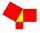Calculate to the nearest tenth cm leg length in the right-angled triangle with hypotenuse length 9 cm and 7 cm long leg.
• Is right?Is a triangle with sides 51, 56, and 77 right triangle?
• Rhombus ABCDRhombus ABCD, |AC| = 90 cm, |BD| = 49 cm. Calculate the perimeter of the rhombus ABCD.
• Two parallel chordsIn a circle 70 cm in diameter, two parallel chords are drawn so that the center of the circle lies between the chords. Calculate the distance of these chords if one of them is 42 cm long and the second 56 cm.
• CathetiThe hypotenuse of a right triangle is 41 and the sum of legs is 49. Calculate the length of its legs.
• Is right?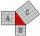Determine whether the triangle with legs (catheti) 19.5 cm and 26 cm and length of the hypotenuse 32.5 cm is rectangular?
• Right isoscelesCalculate area of the isosceles right triangle which perimeter is 26 cm.
• Leg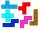Determine the trapezoid area with bases 71 and 42, and the height is 4 shorter than its leg.
• OPT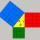What is the perimeter of a right triangle with the legs 14 cm and 21 cm long?
• CathetiOne of the catheti of the right triangle has a length of 12 cm. At what distance from the center of the hypotenuse another cathetus?
• Height UTHow long is height in the equilateral triangle with a side b = 43?
• RectangleThe rectangle is 21 cm long and 38 cm wide. Determine the radius of the circle circumscribing rectangle.
• Right triangleLegs of the right triangle are in the ratio a:b = 2:8. The hypotenuse has a length of 87 cm. Calculate the perimeter and area of the triangle.
• Diagonal - simple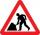Calculate the length of the diagonal of a rectangle with dimensions 5 cm and 12 cm.
• TangentsTo circle with a radius of 41 cm from the point R guided two tangents. The distance of both points of contact is 16 cm. Calculate the distance from point R and circle centre.
• HypotenuseCalculate the length of the hypotenuse of a right triangle if the length of one leg is 4 cm and its content area is 16 square centimeters.
• ArmCalculate the length of the arm r of isosceles triangle ABC, with base |AB| = 14 cm and a height v=18 cm.